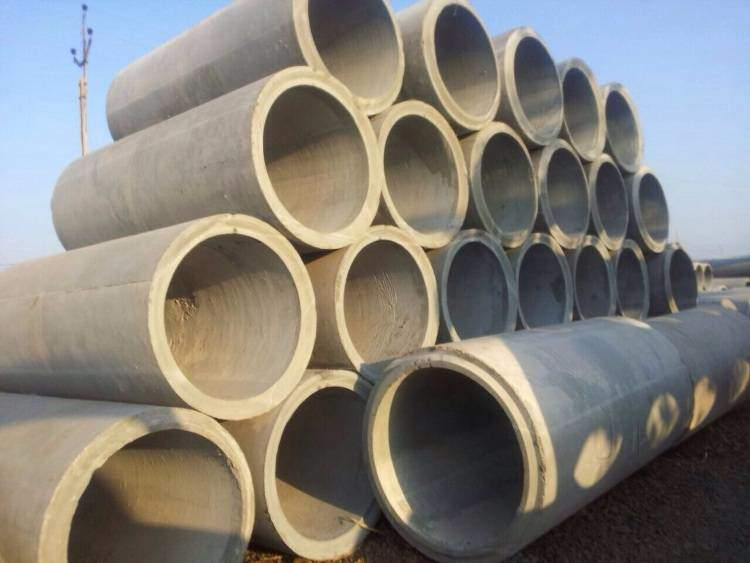# Concrete Tube Calculator  IS : 458/2003

##Concrete Tube Calculation

meter
cm
meter
cm
meter
cm

#### Total Area of Concrete Tube

0.18 m3 | 6.24 ft3

Sr. Material Quantity
1Cement 1 Bags
2Sand 0.11 Ton
3Aggregate 0.20 Ton

## Concrete Tube Calculation

#### Tube Inner Area

$Inner Area ={\mathrm{\pi r}}^{2}$

$r =\frac{\mathrm{Diameter}}{2}$

$r =\frac{\mathrm{0.40}}{2}$

$r =\mathrm{0.20}$

$Inner Area =3.14×\mathrm{0.20}×\mathrm{0.20}$

$Inner Area =\mathrm{0.13}$

#### Tube Outer Area

$Outer Area ={\mathrm{\pi r}}^{2}$

$r =\frac{\mathrm{Diameter}}{2}$

$r =\frac{\mathrm{0.50}}{2}$

$r =\mathrm{0.25}$

$Outer Area =3.14×\mathrm{0.25}×\mathrm{0.25}$

$Outer Area =\mathrm{0.20}$

#### Tube Total Area

$Area=\mathrm{Outer Tube Area - Inner Tube Area}$

$Area =\mathrm{0.13}-\mathrm{0.20}=\mathrm{0.07}$

$Volume=\mathrm{Area}×\mathrm{Height}×\mathrm{No of Column}$

$Volume=\mathrm{0.07}×2.\mathrm{50}×1$

$Volume=0.18{m}^{3}\mathrm{or}6.24{\mathrm{ft}}^{3}$

$Dry Volume=0.18×\mathrm{1.524}$

$Dry Volume=0.27$

#### Cement Volume

$=\frac{\mathrm{Dry Volume}×\mathrm{Cement}}{\mathrm{Sum of Ratio}}$

$=\frac{\mathrm{0.27}×1}{\mathrm{5.5}}$

$=\mathrm{0.05}$

#### No. of Cement Bags

$=\frac{\mathrm{Cement Volume}}{0.035}$

$=\frac{\mathrm{0.05}}{0.035}$

$=\mathrm{2 Bags}$
Note: 1 Bag of cement = 0.035 m3.
1 Cement bag contains = 50 kg cement

#### Sand Volume

$=\frac{\mathrm{Dry Volume}×\mathrm{Sand}}{\mathrm{Sum of Ratio}}$

$=\frac{\mathrm{0.27}×\mathrm{1.5}}{\mathrm{5.5}}$

$=\mathrm{0.07}$

#### Sand in Ton

$=\frac{\mathrm{Sand Volume}×1550}{1000}$

$=\frac{\mathrm{0.07}×1550}{1000}$

$=\mathrm{0.11 Tons}$
Note: By considering dry loose bulk density of sand = 1550 kg/m3.
1000 kg = 1 Ton

#### Aggregate Volume

$=\frac{\mathrm{Dry Volume}×\mathrm{Aggregate}}{\mathrm{Sum of Ratio}}$

$=\frac{\mathrm{0.27}×3}{\mathrm{5.5}}$

$=\mathrm{0.15}$

#### Aggregate in Ton

$=\frac{\mathrm{Aggregate Volume}×1350}{1000}$

$=\frac{\mathrm{0.15}×1350}{1000}$

$=\mathrm{0.20 Tons}$
Note: By considering dry loose bulk density of aggregate = 1350 kg/m3.
1000 kg = 1 Ton

## What is concrete tube calculation?

Reinforced Cement Concrete (RCC) Tube (Pipes) are generally used for water drainage,sewerage,culverts and irrigation. RCC Tube are very much preferred for such usage because they are leak proof, are easily repairable and are non-reactive to sewerage toxins. Concrete Tubes has been estimated to easily last around 100 years and as such they are fit and forget solution which is why they are preferred over other materials for such usage.

• Class : Light duty- NP2, Medium Duty- NP3, Heavy Duty- NP4
• Density : Optimum water cement ratio and High density.
• Length : 2 & 2.5 meter and as per requirement
• Diameter Of RCC Tube (Pipe) : 80, 100, 150. 200, 225, 250, 300, 350, 400, 450, 500, 600, 700, 800, 900, 1000,1100, 1200, 1400, 1600 & 1800.
• Joints : Socket and Spigot, Collar Joints and Flush Joints
• RCC Tube (Pipes) are made from cement, course and fine aggregate, sand, mild steel and HT Rods and bars.##### Concrete Tube Calculation
$Radius =\frac{\mathrm{Diameter}}{2}$

$Area of Inner Tube ={\mathrm{\pi × Inner Radius}}^{2}$

$Area of Outer Tube ={\mathrm{\pi × Outer Radius}}^{2}$

$Total Area of Tube ={\mathrm{\left(Outer Radius - Inner Radius\right)× Height × No of Tube}}^{}$

$Dry Volume =\mathrm{Toatl Area \left(Cubic Meter\right)}×1.524$

$Cement Volume =\frac{\mathrm{Dry Volume}×\mathrm{Cement}}{\mathrm{Sum of Ratio}}$

$No. of Cement Bags =\frac{\mathrm{Cement Volume}}{0.035}$

$Sand Volume =\frac{\mathrm{Dry Volume}×\mathrm{Sand}}{\mathrm{Sum of Ratio}}$

$Sand in Ton=\frac{\mathrm{Sand Volume}×1550}{1000}$

$Aggregate Volume =\frac{\mathrm{Dry Volume}×\mathrm{Aggregate}}{\mathrm{Sum of Ratio}}$

$Aggregate in Ton=\frac{\mathrm{Aggregate Volume}×1350}{1000}$
Where,
• 1.524 is dry volume for the concrete
• 0.035 is volume of one cement bag.
• 1550 is density volume conversion sand m3 to kg.
• 1350 is density volume conversion aggregate m3 to kg.## What are the importance of concrete tube?

Concrete Tube usually for low-pressure applications such as gravity flow or drainage. Pipes for sewage are still predominantly made from concrete or vitrified clay. Reinforced concrete can be used for large-diameter concrete pipes. This pipe material can be used in many types of construction, and is often used in the gravity-flow transport of storm water. Usually such pipe will have a receiving bell or a stepped fitting, with various sealing methods applied at installation.